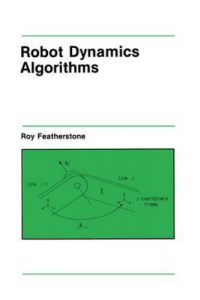اسم المؤلف
Roy Featherstone , David Orin
التاريخ
7 يناير 2020
التصنيف
المشاهدات
التقييم(لا توجد تقييمات)Loading...

بحث بعنوان
Robot Dynamics – Equations and Algorithms
Roy Featherstone
Department of Computer Science
University of Wales, Aberystwyth
Penglais, Aberystwyth SY23 3DB, Wales, UK
David Orin
Department of Electrical Engineering
Ohio State University
Columbus, OH 43210-1272, USA
Abstract
This paper reviews some of the accomplishments in the field of robot dynamics research, from the development of the recursive Newton-Euler algorithm to the present day. Equations and algorithms are given for the most important dynamics computations, expressed in a common notation to facilitate their presentation and comparison.
Conclusion
This paper has reviewed some of the major contributions made in the field of robot dynamics. The main algorithms and associated equations have been given using a common, concise notation. The minimization of computational costs has been key in developing new formulations and algorithms for control and simulation of robotic mechanisms. Roboticists may be credited with developing the most computationallyefficient, low-order algorithms for inverse dynamics, forward dynamics, and the inertia matrix.
Space did not permit us to include extensive discussion of a number of other topics that have been investigated in robot dynamics. These include automatic generation and simplification of symbolic equations of motion, algorithms for parallel computers, and applications to particular classes of systems. For a particular robot configuration, the equations of motion when expressed in symbolic form usually involve the least computation to implement. The use of Kane’s equations  may be of value in this regard, although their use may also involve considerable manipulation of the basic equations to reduce them to the simplest form.
Through the use of parallel algorithms, researchers have been able to reduce the computation of O(N) algorithms to O(log N) on O(N) processors. The application of robot dynamics to multilegged vehicles, biomechanical systems, spacecraft, flexible structures, and underwater robotic vehicles has extended manyof the basic algorithms to the needs of the particular area. Hopefully, as more complex systems and applications are developed, research in robot dynamics will help meet the expanding needs in performance.
كلمة سر فك الضغط : books-world.net